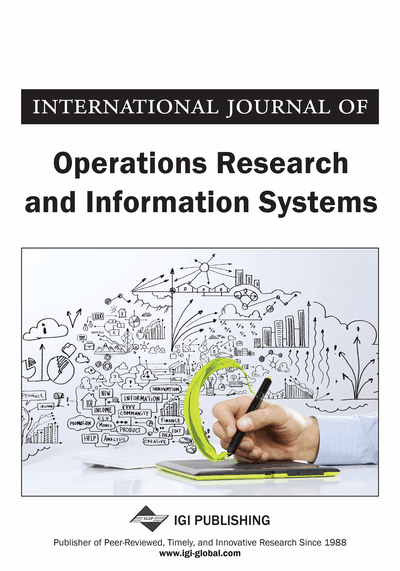# Methods for Solving Fully Fuzzy Transportation Problems Based on Classical Transportation Methods

Amit Kumar (Thapar University, India) and Amarpreet Kaur (Thapar University, India)
DOI: 10.4018/joris.2011100104
Available
\$29.50
No Current Special Offers

## Abstract

There are several methods, in literature, for finding the fuzzy optimal solution of fully fuzzy transportation problems (transportation problems in which all the parameters are represented by fuzzy numbers). In this paper, the shortcomings of some existing methods are pointed out and to overcome these shortcomings, two new methods (based on fuzzy linear programming formulation and classical transportation methods) are proposed to find the fuzzy optimal solution of unbalanced fuzzy transportation problems by representing all the parameters as trapezoidal fuzzy numbers. The advantages of the proposed methods over existing methods are also discussed. To illustrate the proposed methods a fuzzy transportation problem (FTP) is solved by using the proposed methods and the obtained results are discussed. The proposed methods are easy to understand and to apply for finding the fuzzy optimal solution of fuzzy transportation problems occurring in real life situations.
Article Preview
Top

## Introduction

In today's highly competitive market, the pressure on organizations to find better ways to create and deliver value to customers becomes stronger. How and when to send the products to the customers in the quantities, they want in a cost-effective manner, become more challenging. Transportation models provide a powerful framework to meet this challenge. They ensure the efficient movement and timely availability of raw materials and finished goods. The basic transportation problem was originally developed by Hitchcock (1941). The transportation problems can be modeled as a standard linear programming problem, which can then be solved by the simplex method. However, because of its very special mathematical structure, it was recognized early that the simplex method applied to the transportation problem can be made quite efficient in terms of how to evaluate the necessary simplex method information (variable to enter the basis, variable to leave the basis and optimality conditions). Charnes and Cooper (1954) developed the stepping stone method which provides an alternative way of determining the simplex method information. Dantzig and Thapa (1963) used the simplex method to the transportation problem as the primal simplex transportation method. An initial basic feasible solution for the transportation problem can be obtained by using the north-west corner rule, row minima, column minima, matrix minima (least-cost), or the Vogel's approximation method. The modified distribution method is useful for finding the optimal solution for the transportation problem.

In conventional transportation problems it is assumed that decision maker is sure about the precise values of transportation cost, availability and demand of the product. In real world applications, all the parameters of the transportation problems may not be known precisely due to uncontrollable factors. This type of imprecise data is not always well represented by random variable selected from a probability distribution. Fuzzy number (1965) may represent this data. So, fuzzy decision making method is needed here.

Zimmermann (1978) showed that solutions obtained by fuzzy linear programming are always efficient. Subsequently, Zimmermann's fuzzy linear programming has developed into several fuzzy optimization methods for solving the transportation problems. Oheigeartaigh (1982) proposed an algorithm for solving transportation problems where the capacities and requirements are fuzzy sets with linear or triangular membership functions. Chanas et al. (1984) presented a fuzzy linear programming model for solving transportation problems with crisp cost coefficients and fuzzy supply and demand values. Chanas and Kuchta (1996) proposed the concept of the optimal solution for the transportation problem with fuzzy coefficients expressed as fuzzy numbers, and developed an algorithm for obtaining the optimal solution. Saad and Abbas (2003) discussed the solution algorithm for solving the transportation problem in fuzzy environment.

Liu and Kao (2004) described a method for solving fuzzy transportation problems based on extension principle. Gani and Razak (2006) presented a two stage cost minimizing fuzzy transportation problem in which supplies and demands are trapezoidal fuzzy numbers. A parametric approach is used to obtain a fuzzy solution and the aim is to minimize the sum of the transportation costs in the two stages. To deal with uncertainties of supply and demand parameters, Gupta and Mehlawat (2007) transformed the past data pertaining to the amount of supply of the ith supply point and the amount of demand of the jth demand point using level () interval-valued fuzzy numbers.

Dinagar and Palanivel (2009) investigated fuzzy transportation problem, with the aid of trapezoidal fuzzy numbers and proposed fuzzy modified distribution method to find the optimal solution in terms of fuzzy numbers. Pandian and Natarajan (2010) proposed a new algorithm namely, fuzzy zero point method for finding a fuzzy optimal solution for a fuzzy transportation problems, where the transportation cost, supply and demand are represented by trapezoidal fuzzy numbers.

## Complete Article List

Search this Journal:
Reset
Volume 14: 1 Issue (2023): Forthcoming, Available for Pre-Order
Volume 13: 2 Issues (2022)
Volume 12: 4 Issues (2021)
Volume 11: 4 Issues (2020)
Volume 10: 4 Issues (2019)
Volume 9: 4 Issues (2018)
Volume 8: 4 Issues (2017)
Volume 7: 4 Issues (2016)
Volume 6: 4 Issues (2015)
Volume 5: 4 Issues (2014)
Volume 4: 4 Issues (2013)
Volume 3: 4 Issues (2012)
Volume 2: 4 Issues (2011)
Volume 1: 4 Issues (2010)
View Complete Journal Contents Listing